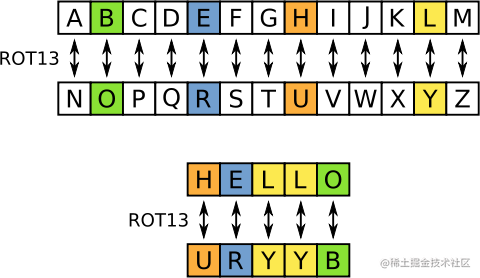# 方法和接口模块

## Stringer

### 解答

``````package main

import "fmt"
import "strings"

func (ip IPAddr) String() string {
s := make([]string, 0, len(ip))
for _, v := range ip {
s = append(s, fmt.Sprintf("%d", v))
}
return strings.Join(s, ".")
}

func main() {
"loopback":  {127, 0, 0, 1},
"googleDNS": {8, 8, 8, 8},
}
for name, ip := range hosts {
fmt.Printf("%v: %v\n", name, ip)
}
}

``````return fmt.Sprintf("%d.%d.%d.%d", ip, ip, ip, ip)

## 错误

### 题目简述

``````type ErrNegativeSqrt float64

``````func (e ErrNegativeSqrt) Error() string

### 解答

``````package main

import (
"fmt"
"math"
)

type ErrNegativeSqrt float64

func (e ErrNegativeSqrt) Error() string {
return fmt.Sprint("cannot Sqrt negative number: ", float64(e))
}

func Sqrt(x float64) (float64, error) {
if x < 0 {
return x, ErrNegativeSqrt(x)
}
if x == 0 {
return 0, nil
}
z := x / 2
d := 1.0
for math.Abs(d) > 1e-15 {
d = (z*z - x) / (2 * z)
z -= d
}
return z, nil
}

func main() {
if v, err := Sqrt(-0.1); err != nil {
fmt.Println(err)
} else {
fmt.Println(v)
}
}

``````return fmt.Sprintf("cannot Sqrt negative number: %g", e)

### 题目简述

`io.Reader` 接口需要一个 `Read` 方法：

``````func (T) Read(b []byte) (n int, err error)

### 解答

``````package main

func (MyReader) Read(b []byte) (int, error) {
b = 'A'
return 1, nil
}

func main() {
}

``````func (MyReader) Read(b []byte) (int, error) {
for i := range b {
b[i] = 'A'
}
return len(b), nil
}

### 题目简述

rot13 是一种简单的替换密码，它将字母表中前13个字母和后13个字母一一对应进行相互替换。编码和解码是相同的操作。wikipedia ROT13

### 解答

``````package main

import (
"io"
"os"
"strings"
"unicode"
)

type rot13Reader struct {
}

func (rot *rot13Reader) Read(b []byte) (int, error) {
n, err := rot.r.Read(b)
for i := range b {
if unicode.IsLetter(rune(b[i])) {
if b[i] >= 'A' && b[i] < 'N' || b[i] >= 'a' && b[i] < 'n' {
b[i] += 13
} else {
b[i] -= 13
}
}
}
return n, err
}

func main() {
s := strings.NewReader("Lbh penpxrq gur pbqr!")
io.Copy(os.Stdout, &r)
}

## 图像

### 题目简述

``````type Image interface {
ColorModel() color.Model
Bounds() Rectangle
At(x, y int) color.Color
}

`Bounds` 应当返回一个 `image.Rectangle` ，例如 `image.Rect(0, 0, w, h)`

`ColorModel` 应当返回 `color.RGBAModel`

`At` 应当返回一个颜色。上一个图片生成器的值 `v` 对应于此次的 `color.RGBA{v, v, 255, 255}`

### 解答

``````package main

import (
"golang.org/x/tour/pic"
"image"
"image/color"
)

type Image struct {
w, h int
}

func (img Image) Bounds() image.Rectangle {
return image.Rect(0, 0, img.w, img.h)
}

func (Image) ColorModel() color.Model {
return color.RGBAModel
}

func (Image) At(x, y int) color.Color {
v := (x + y) / 2
return color.RGBA{uint8(v), uint8(v), 255, 255}
}

func main() {
m := Image{256, 256}
pic.ShowImage(m)
}

`At` 方法定义了每一个位置的像素是什么颜色。你可以像上一个图片生成器一样，通过更改 `v` 的公式来生成不同的图案；或者你也可以更改 `color.RGBA` 的值来进行更自由的创作。